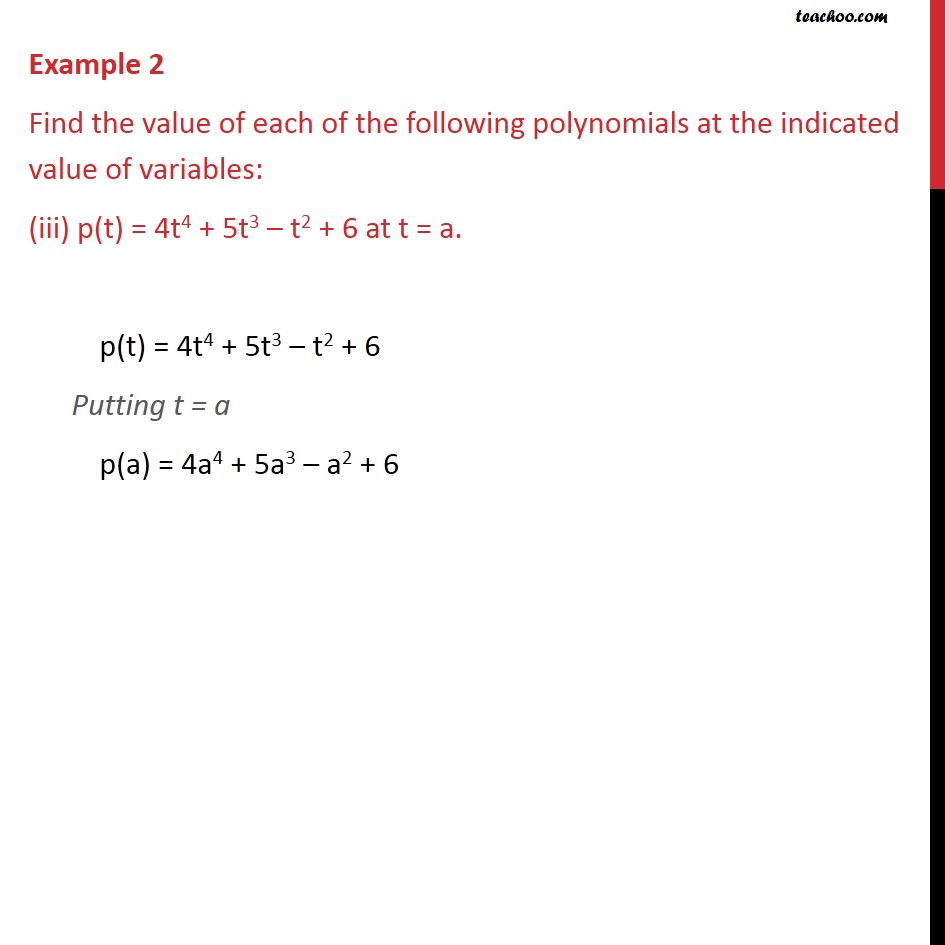Examples

Chapter 2 Class 9 Polynomials
Serial order wiseLearn in your speed, with individual attention - Teachoo Maths 1-on-1 Class

### Transcript

Example 2 Find the value of each of the following polynomials at the indicated value of variables: (iii) p(t) = 4t4 + 5t3 – t2 + 6 at t = a. p(t) = 4t4 + 5t3 – t2 + 6 Putting t = a p(a) = 4a4 + 5a3 – a2 + 6